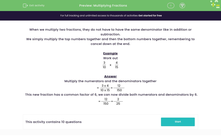# Multiply Fractions

In this worksheet, students will multiply two simple fractions.Key stage:  KS 3

Curriculum topic:   Number

Curriculum subtopic:   Use Four Operations for All Numbers

Difficulty level:#### Worksheet Overview

When we multiply two fractions together, they do not have to have the same denominator as they do in addition or subtraction.

We simply multiply the top numbers together and then the bottom numbers together, remembering to cancel down at the end.

Example

Work out:

 3 x 4 10 15

Multiply the numerators and the denominators together:

 = 3 x 4 = 12 10 x 15 150

This new fraction has a common factor of 6, so we can now divide both numerators and denominators by 6:

 = 12 = 2 150 25

Let's have a go at some questions now.

### What is EdPlace?

We're your National Curriculum aligned online education content provider helping each child succeed in English, maths and science from year 1 to GCSE. With an EdPlace account you’ll be able to track and measure progress, helping each child achieve their best. We build confidence and attainment by personalising each child’s learning at a level that suits them.

Get started